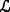Substitutability $$\tilde {\gamma }$$ Expected value $$E[\tilde {\gamma }]/E[\tilde {\beta }]$$
Low(15,35) 25 0.25
Medium(40,60) 50 0.50
High(70,80) 75 0.75